# Texas Go Math Kindergarten Lesson 10.8 Answer Key Compose and Decompose Numbers Up to 10

Refer to our Texas Go Math Kindergarten Answer Key Pdf to score good marks in the exams. Test yourself by practicing the problems from Texas Go Math Kindergarten Lesson 10.8 Answer Key Compose and Decompose Numbers Up to 10.

## Texas Go Math Kindergarten Lesson 10.8 Answer Key Compose and Decompose Numbers Up to 10

Essential Question
How can you solve problems using a picture?

Unlock the Problem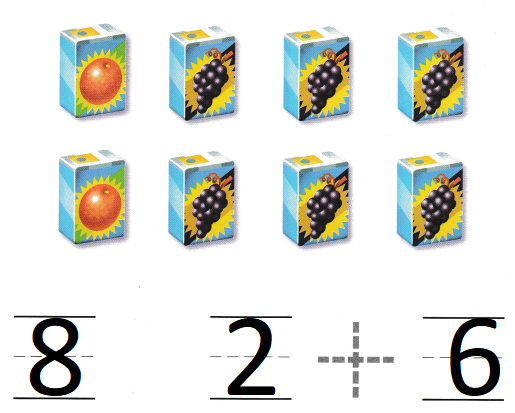Explanation:
There are some juice boxes for snack. Two of the juice boxes are orange juice.
Six of the juice boxes are grape juice
2 + 6 = 8
8 juices are altogether

Directions
There are some juice boxes for snack. Two of the juice boxes are orange juice. Six of the juice boxes are grape juice. How many juice boxes are there altogether? Use the picture to solve the problem. Write the numbers and trace the symbol.

Try Another Problem

Question 1.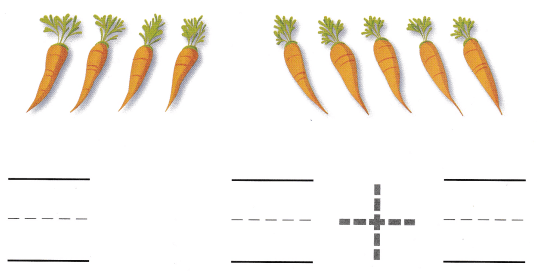Explanation:
Monica has some carrots. She ate 4 carrots for snack.
She ate 5 carrots for lunch.
4 + 5 = 9
so, Monica eat 9 carrots in all

Question 2.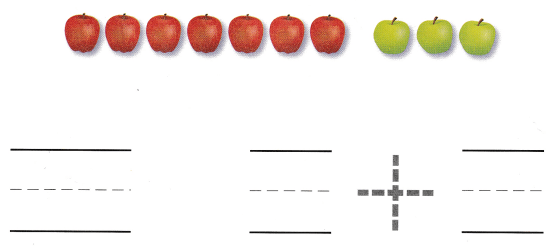Explanation:
Seven apples are red. Three apples are green
7 + 3 = 10
10 apples are there in all.

Directions
1. Monica has some carrots. She ate 4 carrots for snack. She ate 5 carrots for lunch. How many carrots did Monica eat? Use the picture to solve the problem. Write the numbers and trace the symbol. 2. Some children brought in apples for snack. Seven apples are red. Three apples are green. How many apples are there? Use the picture to solve the problem. Write the numbers and trace the symbol.

Share and Show

Question 3.Explanation:
There are six bananas on the snack table.
Two bananas are green
6 – 2 = 4
4 bananas are yellow

Question 4.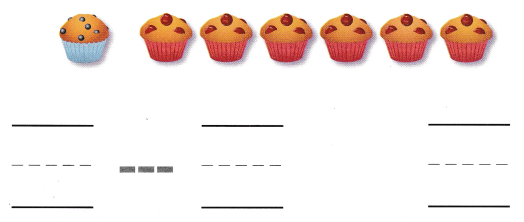Explanation:
Seven muffins are on the snack table.
One is a blueberry muffin and the rest are cherry muffins.
7 – 1 = 6
6 are cherry muffins.

Directions
3. There are six bananas on the snack table. Two bananas are green. How many bananas are yellow? Use the picture to solve the problem. Write the numbers and trace the symbol. 4. Seven muffins are on the snack table. One is a blueberry muffin and the rest are cherry muffins. How many are cherry muffins? Use the picture to solve the problem. Write the numbers and trace the symbol.

Home Activity

• Have your child draw a set of ten or fewer balloons. Have him or her circle and mark on X on some balloons to show that they have popped. Then have your child tell a word problem to match.

Question 5.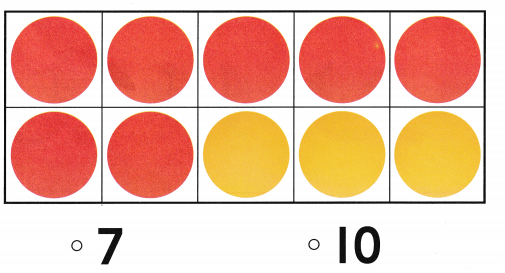Explanation:
There are 10 counters,
if you take apart 3
then 10 – 3 = 7
7 red counters are remaining.

Question 6.Explanation:
There are 7 blocks out of which 5 are blue
so, 7 – 5 = 2
2 are red blocks.

Question 7.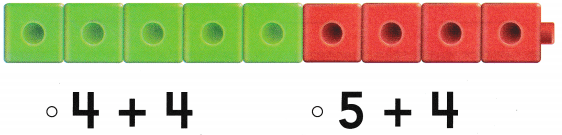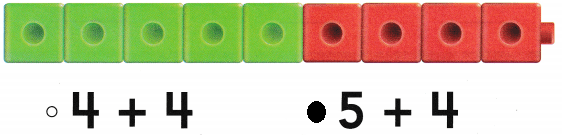Explanation:
5 green blocks and 4 red blocks
so, 5 + 4 = 9
There are 9 blocks.

Directions
5. There are 10 counters, How many counters will be left if you take apart 3? 6-7. Which does the model show?

### Texas Go Math Kindergarten Lesson 10.8 Homework and Practice

Question 1.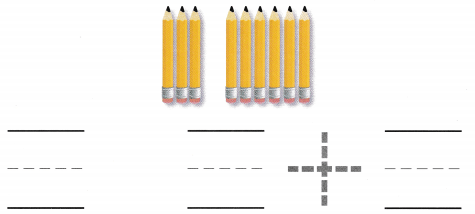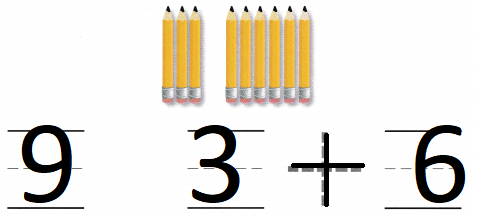Explanation:
Carolyn had some pencils. She gave 3 pencils to her brother.
She gave 6 pencils to her sister.
so, 3 + 6 = 9
there are 9 pencils.

Question 2.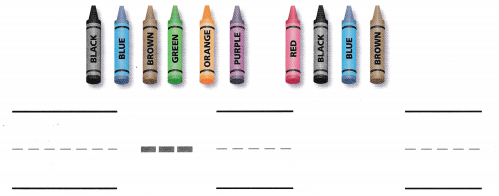Explanation:
There are 10 crayons in the classroom.
Six crayons are used for art class.
so, 10 – 6 = 4
4 crayons are left.

Directions
1. Carolyn had some pencils. She gave 3 pencils to her brother. She gave 6 pencils to her sister. How many pencils did Carolyn have? Use the picture to solve the problem. Write the numbers and trace the symbol. 2. There are 10 crayons in the classroom. Six crayons are used for art class. How many crayons are left? Use the picture to solve the problem. Write the numbers and trace the symbol.

Texas Test Prep

Question 3.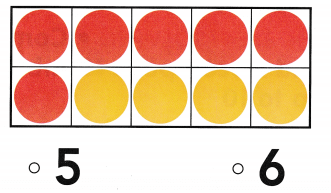Explanation:
There are 10 counters.
you have to take away 4
10 – 4 = 6
6 red counters.

Question 4.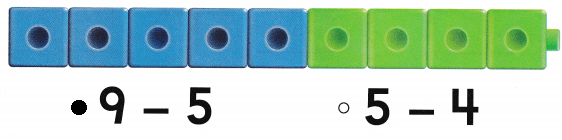Explanation:
There are 9 blocks
5 are blue blocks
so, 9 – 5 = 4
4 are green blocks

Question 5.